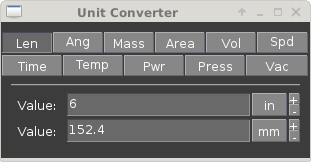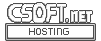Agar 1.7 Manual

(Printable Version)
AG_Units(3)

# SYNOPSIS

```#include <agar/core.h>
#include <agar/gui.h>
```

# DESCRIPTIONThe Agar AG_Units interface implements unit conversion. The AG_Unit structure is defined as follows:
```typedef struct ag_unit {
char *key;
char *abbr;
char *name;
double divider;
double (*func)(double, int mode);
} AG_Unit;
```

The key member is a unique identifier for this unit. abbr is an abbreviated symbol, and name is the full name of the unit.

The linear conversion factor is specified in divider. For non-linear conversions, func can be defined instead (the first argument to the function is the value to convert; if mode is 1, the function make the reverse conversion).

A set of standard conversion unit groups are defined in gui/units.c in the Agar sources. The standard groups are summarized below.

# GENERAL ↑

 agPercentageUnits Value in percentile agFrequencyUnits Units of frequency agTimeUnits Units of time

# LENGTHS & ANGLES ↑

 agLengthUnits Units of length/distance agAngleUnits Units of angular measurement agVideoUnits Units of video pixel resolution agAreaUnits Units of area agVolumeUnits Units of volume agSpeedUnits Units of velocity

# PHYSICAL ↑

 agMassUnits Units of weight agPressureUnits Units of pressure and stress (general) agVacuumUnits Units of pressure (low pressures) agThermalConductivityUnits Units of thermal conductivity agThermalExpansionUnits Units of thermal expansion agDensityUnits Units of density agLightUnits Units of light intensity agSubstanceAmountUnits Units of substance amount (moles)

# THERMODYNAMICS ↑

 agPowerUnits Units of power agTemperatureUnits Units of temperature agEnergyPerSubstanceAmountUnits Units of energy per substance amount agMolarHeatCapacityUnits Units of molar heat capacity

# ELECTRICAL ↑

 agEMFUnits Units of electromotive force / voltage agCurrentUnits Units of electrical current agResistanceUnits Units of electrical resistance agResistanceTC1Units Units of first-order temperature coefficients agResistanceTC2Units Units of second-order temperature coefficients agResistivityUnits Units of resistivity of a material agCapacitanceUnits Units of electrical capacitance agInductanceUnits Units of electrical inductance

# INTERFACE ↑

const AG_Unit * AG_FindUnit (const char *key)

const AG_Unit * AG_BestUnit (const AG_Unit *unit_group, double n)

char * AG_UnitFormat (double n, const AG_Unit unit_group[])

const char * AG_UnitAbbr (const AG_Unit *unit)

double AG_Unit2Base (double n, const AG_Unit *unit)

double AG_Base2Unit (double n, const AG_Unit *unit)

double AG_Unit2Unit (double n, const AG_Unit *unit_from, const AG_Unit *unit_to)

The AG_FindUnit() function searches the unit database for a unit matching the given key, and returns a pointer to the unit on success or NULL if none was found.

The AG_BestUnit() function returns the unit expected to yield the least number of non-significant figures when formatting the given number n. AG_UnitFormat() formats the given number n using the best unit in unit_group.

AG_UnitAbbr() returns the abbreviation string associated with the given unit.

The AG_Unit2Base() function converts from n in specified units to the equivalent number of base units. AG_Base2Unit() converts n base units to the equivalent number of specified units.

# EXAMPLES ↑

One widget which uses this interface is AG_Numerical(3), which accepts unit arguments. The following code fragment creates a widget for editing a length value given in meters:
```float length = 1.234;
AG_Numerical *num;
num = AG_NumericalNewFlt(parent, 0, "m", "Length: ", &length)
```

The following code fragment prints the equivalent milliseconds for a given n number of seconds:
```printf("%f seconds = %f milliseconds", n,
AG_Base2Unit(n, AG_FindUnit("ms")));
```

The following code fragment prints the equivalent of 27 degrees Celsius, in kilo Kelvins:
```const AG_Unit *degC = AG_FindUnit("degC");
const AG_Unit *kk = AG_FindUnit("kk");

printf("27C = %fkk", AG_Unit2Unit(27.0, degC, kk));
```

This code fragment displays the value of r using the resistance unit most suitable to its magnitude.
```printf("Resistance = %s", AG_UnitFormat(r, agResistanceUnits));
```

Also see tests/unitconv.c in the Agar source distribution.